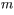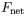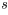# Problem: The kinetic energy K of an object of mass m moving at a speed v is defined as K = (1/2)mv2. It seems reasonable to say that the speed of an object--and, therefore, its kinetic energy--can be changed by performing work on the object. In this problem, we will explore the mathematical relationship between the work done on an object and the change in the kinetic energy of that object.Let us now consider the situation quantitatively. Let the mass of the sled be  and the magnitude of the net force acting on the sled be. The sled starts from rest.Consider an interval of time during which the sled covers a distance and the speed of the sled increases from v1 to v2. We will use this information to find the relationship between the work done by the net force (otherwise known as the net work) and the change in the kinetic energy of the sled.A)Find the net force Fnet acting on the sled.Express your answer in terms of some or all of the variables m,s,v1, and v2.B)Find the net work Wnet done on the sled.Express your answer in terms of some or all of the variables Fnet and s.

###### FREE Expert Solution

Work:

$\overline{){\mathbf{W}}{\mathbf{=}}{\mathbf{F}}{\mathbf{·}}{\mathbf{d}}}$

A)

In this case, F = Fnet and d = s, the distance.

From the law of conservation of energy, the work done is equal to the change in kinetic energy.

${\mathbf{W}}{\mathbf{=}}\frac{\mathbf{1}}{\mathbf{2}}{\mathbf{m}}{\mathbf{\left(}}{{{\mathbf{v}}}_{{\mathbf{2}}}}^{{\mathbf{2}}}{\mathbf{-}}{{{\mathbf{v}}}_{{\mathbf{1}}}}^{{\mathbf{2}}}{\mathbf{\right)}}$

93% (22 ratings)###### Problem Details

The kinetic energy K of an object of mass m moving at a speed v is defined as K = (1/2)mv2. It seems reasonable to say that the speed of an object--and, therefore, its kinetic energy--can be changed by performing work on the object. In this problem, we will explore the mathematical relationship between the work done on an object and the change in the kinetic energy of that object.

Let us now consider the situation quantitatively. Let the mass of the sled beand the magnitude of the net force acting on the sled be. The sled starts from rest.

Consider an interval of time during which the sled covers a distanceand the speed of the sled increases from v1 to v2. We will use this information to find the relationship between the work done by the net force (otherwise known as the net work) and the change in the kinetic energy of the sled.

A)

Find the net force Fnet acting on the sled.

Express your answer in terms of some or all of the variables m,s,v1, and v2.

B)

Find the net work Wnet done on the sled.

Express your answer in terms of some or all of the variables Fnet and s.